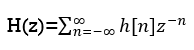Goseeko blog# What is z-Transform?

The most important use of z-transform is its ability to completely characterize signals and linear systems in the most general ways possible. The z-transform is primarily used to convert the discrete time domain signal into discrete frequency domain signal. The z-transform for LTI system is defined asDue to its convolution property, the z-transform is a powerful tool to analyse LTI systems. From the convolution property Y(z)=H(z)X(z).

Where:

Y(z)= z-transform of system output

H(z) = z-transform of impulse response

X(z)= z-transform of system input

A discrete time LTI system is causal if and only if the ROC of its system function is exterior of the circle including infinity. The ROC is the exterior of the outermost pole. H(z) is the ratio of polynomials in z, the order of the numerator cannot be greater than the order of the denominator.

The LTI system is stable if and only if the ROC of the system function H(z) includes the unit circle |Z|=1. If it is a causal system it will be stable if and only if all the poles of H(z) lie inside the unit circle.

## Region of Convergence

The range of variation of z for which z-transform converges is called the region of convergence of z-transform.

Properties of ROC of z-Transform

• ROC does not contain any poles.
• If x(n) is a finite sequence and x(z) converges for some value of z, then ROC can be the entire z plane except at z=0 and ∞.
• As x(n) is a right sided sequence and x(z) converges for some value of z, then the ROC is the of the form |z|>Rmax where Rmax is the radius.
• If x(n) is left sided sequence and x(z) converges for some value of z then the ROC is of the form |z|<Rmin where Rmin  is the smallest magnitude of any pole of x(z). Thus, the ROC is the entire circle having Rmin.
• If x(n) is a double sided sequence and x(z) converges for some value of z then the ROC is of the form.

R1 <|z| < R2

Rmin < |z| <Rmax. Where R1, R2 are the magnitude of two poles of x(z)

## Properties of z-transform for Causal Signals

Linearity

If X1(z) = Z{x1(n)} and X2(z) = Z{x2(n)}

Then

Z{ax1(n) + bx2(n)} = a X1(z) + b X2(z)

Time Shifting

If X(z) = Z{x(n)} and initial conditions for x(n) are zero then

Z{x(n-m) } = z-m X(z)

Where m is a positive integer.

Multiplication by exponential sequence

If X(z ) = Z{x(n)} then

Z [ an x(n) ] = X(a-1 z)

Convolution

We know that

Z{x(n) * h(n) } = X(z) H(z)

Multiplication by n

If Z{x(n)} = X(z) then

Z{n x(n)} = -z d/dz X(z)

Interpretation of stability in z-domain

One of the most important characteristics of the z-plane is that the region of filter stability is mapped to the inside of the unit circle on the z-plane. Given the H(z) transfer function of a digital filter, we can examine that function’s pole locations to determine filter stability. If all poles are located inside the unit circle, the filter will be stable. On the other hand, if any pole is located outside the unit circle, the filter will be unstable.

Interested in learning about similar topics? Here are a few hand-picked blogs for you!